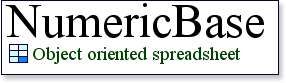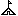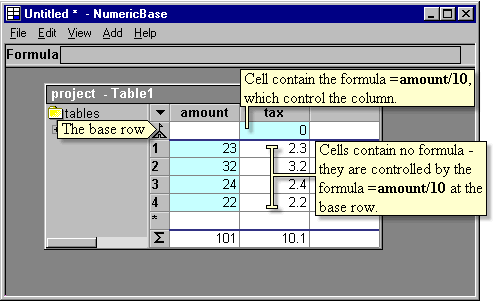# The base row

Single formula controls a column
A Formula located at the base row (the row labeled with the "base" icon), controls all the cells at the same column. In the following screenshot, the formula =amount/10 in the tax column sets the values of the cells across the column to be 10 percent of the corresponding values from the amount column.Screenshot: the formula =amount/10 controls the values of the tax column.

Note that the formula =amount/10 is the only formula at the column - all the other cells are controlled by this formula.